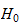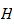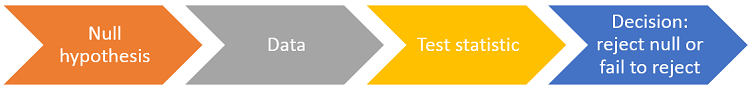StatLect

# Null hypothesis

In a test of hypothesis, a sample of data is used to decide whether to reject or not to reject a given hypothesis about the probability distribution from which the sample was extracted. This hypothesis is called null hypothesis or simply "the null".## Symbol

The null hypothesis is usually denoted by the symbol(read "H-zero", "H-nought" or "H-null"). The letterin the symbol stands for "Hypothesis".

## The null is like the defendant in a criminal trial

Formulating null hypotheses and subjecting them to statistical testing is one of the workhorses of the scientific method. Scientists in all fields make conjectures about the phenomena they study, translate them into null hypotheses and gather data to test them. This process resembles a trial:

• the defendant (the null hypothesis) is accused of being guilty (wrong);

• evidence (data) is gathered in order to prove the defendant guilty (reject the null);

• if there is evidence beyond any reasonable doubt, the defendant is found guilty (the null is rejected);

• otherwise, the defendant is found not guilty (the null is not rejected).

The reader is advised to keep this analogy in mind because it helps to better understand statistical tests, their limitations, use and misuse and frequent misinterpretation.

## How is the null hypothesis tested?

Before collecting the data:

1. we decide how to summarize the relevant characteristics of the sample data in a single number, the so-called test statistic (note that before being collected the data is regarded as random, and therefore the test statistic is a random variable);

2. we derive the probability distribution of the test statistic under the hypothesis that the null is true;

3. we decide what probability of incorrectly rejecting the null we are willing to tolerate (the size of the test);

4. we choose one or more intervals of values (collectively called rejection region) such that the probability that the test statistic falls within these intervals is equal to the desired size;

Then the data is collected and used to compute the value of the test statistic. A decision is taken as follows:

• if the test statistic falls within the rejection region, then the null hypothesis is rejected;

• otherwise, it is not rejected.## Examples

Here are some examples of practical problems that lead to formulate and test a null hypothesis.

### Example 1 - Proportion of defective items

A new method is proposed to produce light bulbs. The proponents claim that it produces less defective bulbs than the method currently in use. In order to check the claim, we can set up a statistical test as follows:

• measurement: we keep light bulbs on for 10 consecutive days, and then we record whether they are still working at the end of the test period;

• null hypothesis: the probability that a light bulb produced with the new method is still working at the end of the test period is the same as that of a light bulb produced with the old method;

• data: 100 light bulbs are tested; 50 of them are produced with the new method (group A); the remaining 50 are produced with the old method (group B); the final data comprises 100 observations of: 1) an indicator variable which is equal to 1 if the light bulb is still working at the end of the test period and 0 otherwise; 2) a categorical variable that records the group (A or B) to which each light bulb belongs;

• test statistic: a z-statistic is computed by: 1) taking the difference between the proportions of working light bulbs in groups A and B (the proportions are estimates of the probabilities of not being defective, which are equal for the two groups under the null hypothesis); 2) subtracting from this difference its expected value (which is zero under the null) and dividing it by its standard deviation (it can be derived analytically); the quantity thus obtained can be approximated by a standard normal distribution (see here for details);

• critical region: we decide that the size of the test must be 5%, that is we are going to tolerate a 5% probability of incorrectly rejecting the null; the critical region is the right 5%-tail of the normal distribution, that is, all values greater than 1.645 (see the glossary entry on critical values if you are wondering how this value was obtained);

• decision: if the test statistic is greater than 1.645, then the null is rejected; otherwise, it is not rejected;

• interpretation: a rejection is interpreted as significant evidence that the new production method produces less defective items; failure to reject is interpreted as insufficient evidence that the new method is better.

### Example 2 - Reliability of a production plant

A production plant incurs high costs when production needs to be halted because some machinery fails. The plant manager has decided he is not willing to tolerate more than one halt per year on average. If the expected number of halts per year is greater than 1, he will make new investments in order to improve the reliability of the plant. A statistical test is set up as follows:

• measurement: the reliability of the plant is measured by the number of halts;

• null hypothesis: the number of halts in a year has a Poisson distribution with expected value equal to 1 (assuming a Poisson distribution is typical in reliability testing);

• data: the manager cannot wait more than one year before taking a decision; there will be a single datum at his disposal: the number of halts observed during one year;

• test statistic: the number of halts is used as a test statistic; by assumption, it has a Poisson distribution under the null;

• critical region: the manager decides that the probability of incorrectly rejecting the null can be at most 10%; a Poisson random variable with expected value equal to 1 takes values larger than 1 with probability 26.42% and larger than 2 with probability 8.03%; therefore, it is decided that the critical region will be the set of all values greater than or equal to 3.

• decision: if the test statistic is strictly greater than or equal to 3, then the null is rejected; otherwise, it is not rejected;

• interpretation: a rejection is interpreted as significant evidence that the production plant is not reliable enough (the average number of halts per year is significantly larger than tolerated); failure to reject is interpreted as insufficient evidence that the plant is unreliable.

## Rejection and failure to reject

This section discusses the main problems that arise in the interpretation of the outcome of a statistical test (reject / not reject).

### Not rejecting and accepting are not the same thing

When the test statistic does not fall within the critical region, then we do not reject the null hypothesis. Does this mean that we accept the null? Not really. In general, failure to reject does not constitute, per se, strong evidence that the null hypothesis is true. Remember the analogy between hypothesis testing and a criminal trial. In a trial, when the defendant is declared not guilty, this does not mean that the defendant is innocent. It only means that there was not enough evidence (not beyond any reasonable doubt) against the defendant. In turn, lack of evidence can be due either 1) to the fact that the defendant is innocent, or 2) to the fact that the prosecution has not been able to provide enough evidence against the defendant, even if the latter is guilty. This is the very reason why courts do not declare defendants innocent, but they use the locution "not guilty". In a similar fashion, statisticians do not say that the null hypothesis has been accepted, but they say that it has not been rejected.

### Failure to reject can be due to lack of power

To better understand why failure to reject does not in general constitute strong evidence that the null hypothesis is true, we need to use the concept of statistical power. The power of a test is the probability (calculated ex-ante, that is, before observing the data) that the null will be rejected when another hypothesis (called alternative hypothesis) is true.

Let's consider the first of the two examples above (the clinical trial). In that example, the null hypothesis is that the 1-year survival probability of patients treated with the new drug is the same as that of patients treated with the old drug. Let's make the alternative hypothesis that the survival probability of patients treated with the new drug is 10% higher than that of patients treated with the old drug (assume that a 10% increase is considered a significant improvement by the medical community). How much is the ex-ante probability of rejecting the null if this alternative hypothesis is true? If this probability (the power of the test) is small, then it is very likely that we will not reject the null even if it is wrong. Going back to the analogy with criminal trials, it means that the prosecution will most likely not be able to provide sufficient evidence, even if the defendant is guilty.

Thus, in the case of lack of power, failure to reject is almost meaningless (it was anyway highly likely). This is why it is good statistical practice to compute the power of a test (against a relevant alternative) before actually performing it. If the power is found to be too small, there are usually remedies. In particular, statistical power can usually be increased by increasing the sample size (see, e.g., the lecture on hypothesis tests about the mean).

### Rejections are easier to interpret, but be careful

As we have explained above, interpreting a failure to reject the null hypothesis is not always straightforward. Instead, interpreting a rejection is somewhat easier. When we reject the null, we know that the data has provided a lot of evidence against the null. In other words, it is unlikely (how unlikely depends on the size of the test) that the null is true given the data we have observed.

There is an important caveat though. The null hypothesis is often made up of several assumptions, including:

• the main assumption (the one we are testing);

• other assumptions (e.g., technical assumptions) that are needed in order to set up the statistical model we use to carry out the hypothesis test.

For instance, in Example 2 above (reliability of a production plant), the main assumption is that the expected number of production halts per year is equal to 1. But there is also a technical assumption: the number of production halts has a Poisson distribution.

It must be kept in mind that a rejection is always a joint rejection of the main assumption and all the other assumptions. Therefore, we should always ask ourselves whether the null has been rejected because the main assumption is wrong or because the other assumptions are violated. In the case of Example 2 above, is a rejection of the null due to the fact that the expected number of halts is greater than 1 or is it due to the fact that the distribution of the number of halts is very different from a Poisson distribution?

When we suspect that a rejection is due to the inappropriateness of some technical assumption (e.g., assuming a Poisson distribution in the example), we say that the rejection could be due to mis-specification of the model. The right thing to do when these kind of suspicions arise is to conduct so-called robustness checks, that is, to change the technical assumptions and carry out the test again. In our example, we could re-run the test by assuming a different probability distribution for the number of halts (e.g., a negative binomial or a compound Poisson - do not worry if you have never heard about these distributions). If we keep obtaining a rejection of the null even after changing the technical assumptions several times, the we can say that our rejection is robust to several different specifications of the model.

## Takeaways - How to (and not to) formulate a null hypothesis

What are the main practical implications of everything we have said thus far? How does the theory above help us to set up and test a null hypothesis? What we said can be summarized in the following guiding principles:

1. A test of hypothesis is like a criminal trial and you are the prosecutor. You want to find evidence that the defendant (the null hypothesis) is guilty. Your job is not to prove that the defendant is innocent. If you find yourself hoping that the defendant is found not guilty (i.e., the null is not rejected) then something is wrong with the way you set up the test. Remember: you are the prosecutor.

2. Compute the power of your test against one or more relevant alternative hypotheses. Do not run a test if you know ex-ante that it is unlikely to reject the null when the alternative hypothesis is true.

3. Beware of technical assumptions that you add to the main assumption you want to test. Make robustness checks in order to verify that the outcome of the test is not biased by model mis-specification.

## More examples

More examples of null hypotheses and how to test them can be found in the following lectures.

Where the example is found Null hypothesis
Hypothesis testing about the mean The mean of a normal distribution is equal to a certain value
Hypothesis testing about the variance The variance of a normal distribution is equal to a certain value
Maximum likelihood - hypothesis testing A vector of parameters estimated by MLE satisfies a set of linear or non-linear restrictions

## More details

The lecture entitled Hypothesis testing provides a more detailed mathematical treatment of null hypotheses and how they are tested.